Subscribe to our newsletter to receive the latest news and events from TWI:

# Plastic Strain and Cleavage Fracture Predictions in Steel

## Effects of Plastic Strain on Cleavage Fracture Predictions in Steel

S. R. Bordet 1,2, A. D. Karstensen 2, C. S. Wiesner 2 and D. M. Knowles 1

1 University of Cambridge, Department of Materials Science and Metallurgy, Pembroke Street, Cambridge, CB2 3QZ, U.K.
2 TWI, Granta Park, Great Abington, Cambridge, CB1 6AL, U.K.

Reprinted from the Proceedings of the 10th International Conference on Fracture, Honolulu, Hawaii, 2-6 December 2001 with permission from Elsevier.

## Abstract

This work analyses the influence of plastic strain on cleavage fracture in a lower bainitic Grade 450EMZ steel. It is shown that a dual stress/plastic strain criterion is needed to describe the conditions of cleavage initiation and early propagation. This serves to explain why inconsistent and/or unrealistic shape factor estimates for stress-only Weibull distributions of cleavage strengths may be often encountered in the literature. The general ability of small-scale yielding expressions to describe the failure probability of toughness specimens is re-explained in terms of the plastic strain evolutions in fracture specimens.

## Introduction

The cumulative failure probability by cleavage is commonly expressed in terms of a Weibull distribution: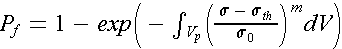(1)

where m, σ0 are, respectively, the shape and scaling factors, σth is a threshold stress below which there is zero probability of failure, V p is the plastic zone size (or some fraction thereof) and σ is generally the maximum principal stress. [1-3] In the particular case of small-scale yielding (SSY), the integration in Eqn. 1 can be solved as a closed-form expression, as shown by several authors. [1-3]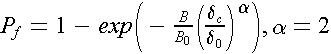(2)

In Eqn. 2, B is the fracture specimen thickness corresponding to the critical Crack Tip Opening Displacement (CTOD) values, δ2, B0 is a reference thickness, α is the shape factor and δ0 is the CTOD value at which a specimen of thickness B0 will have a 63.2% failure probability. Eqn. 2 has the form of a Weibull distribution with a fixed value of 2 for the shape factor α. If expressed in terms of K JC or J-Integral, α equals 4 and 2, respectively. Eqn. 2 can be modified to account for the existence of a threshold toughness δmin: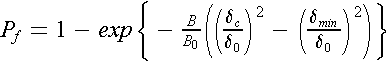(3)

However, Wallin and Anderson et al. [2,3] found that Eqn. 3 did not adequately describe the true fracture behaviour. They suggested [2,3] that a better fit to the δc-results was obtained if P f was arbitrarily re-written as a three-parameter Weibull distribution: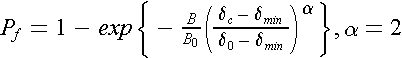(4)

Eqns. 2, 3 and 4 serve as the foundation of many models to quantify size effects and address the inherent probabilistic nature of cleavage fracture. [1-3] Eqn. 4 has been extensively validated on a wide range of steels and forms part of the new ASTM E 1921-97 to describe toughness scatter and size effects. The less flexible Eqn. 2 has also proved to work reasonably well, even if strict SSY conditions are not satisfied.  The good consistency of Eqn. 2 contrasts with the difficulty in establishing typical values for the parameters (often called Weibull parameters) in Eqn. 1: in a recent round-robin, estimates for m were found to vary from 10 to 50 for similar structural steels.  As pointed out by many researchers [1-3] , Eqn. 2 actually predicts that, under SSY conditions, the scatter of fracture toughness is independent of the cleavage initiator distribution. The only material characterizing parameter is J 0, since the scale factor of 2 results from the weakest-link and SSY assumptions. However, it was shown  that an infinite number of possible parameter pairs ( m, δ0) or sets ( m, δ0, δth) in Eqn. 1 lead to the same value of J 0 and this could explain the difficulty in finding consistent m values. Another answer, examined below, is likely to be that the special representation of the failure probability in Eqn. 2, derived from the assumption that the stress field is self-similar in the plastic zone, indirectly addresses the effects of plastic strain on cleavage fracture. The discussion is based on Eqn. 2 rather than Eqn. 4, because Eqn. 4 has no theoretical basis yet. 

## Influence of plastic strain on models predictions

The above proposal has been investigated using CTOD results from a Grade 450EMZ lower bainitic steel plate produced according to BS 7191. The microstructure of Grade 450EMZ is shown in Figure 1. The data sample consisted of eighteen small 10x10 mm 2 (referred to as Set 1) and twenty full-thickness 50x50 mm 2 (referred to as Set 2) Single Edge Notch Bend (SENB) specimens with a nominal crack depth to width ratio a 0/W of 0.5. The small and full-thickness SENB specimens were tested in three point bending, according to BS 7448, at temperatures of -140°C and -130°C respectively. The CTOD results are plotted in Figure 2. All specimens exhibited cleavage fracture without prior ductile tearing.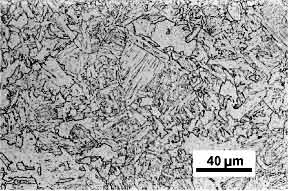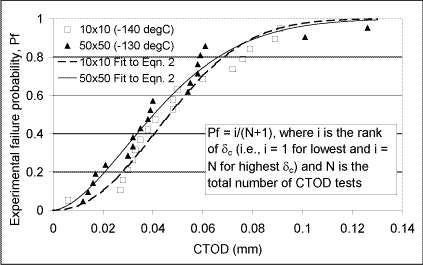Fig. 2. δc-results for the SENB specimens

Fractographic analyses were carried out on a scanning electron microscope (SEM) to investigate the conditions of cleavage initiation. Most specimens of Set 1 and a few specimens of Set 2 were selected for comparison, particularly specimens that fractured at a high or a low CTOD. The main initiation point was determined using river lines patterns and its distance from the crack tip was measured. In all cases, it was found that cleavage initiated at grain boundaries. However, notable differences in the initiation conditions existed for specimens with toughness lying at the extremes of the CTOD values range. In Set 1, the very low toughness of one specimen ( δc = 0.006 mm) is explained by an unexpected early intergranular failure that then changed into transgranular cleavage. For specimens with the highest CTOD values, the initiation points were found to be located closer to the crack tip than in most specimens with lower CTOD, in regions where microductile behaviour (microvoids) began to occur.

Finite element (FE) models for both geometries  were used to infer the original location of the initiation sites in the specimen before loading. For simplicity, the initiation points were assumed to lie in the plane of the fatigue crack. The distance from the crack tip to the peak stress occurring on the crack plane was also determined in the undeformed configuration at different CTOD values. Both distances are plotted against the critical CTOD values for both geometries in Figure 3 (no data point for specimen 2 of Set 1 is plotted, as no clear initiation point could be found in the intergranular fracture zone). Figure 3 clearly shows that initiation points can be divided into two groups with different nucleation conditions: one, containing most results (Group 1), the other one containing only the highest δc-values of the two geometries (Group 2). Group 1 lies above Group 2, expressing the tendency of cleavage nucleating nearer to the crack tip at higher CTOD. Secondly, all initiation points lie below the 'peak stress lines', which indicates that all initiation points were located between the crack tip and the peak stress location. There is also a general tendency for the distances of both groups to increase proportionally with CTOD, or equivalently with peak stress location distances, so that both groups remain at an approximately constant distance from the peak stress position.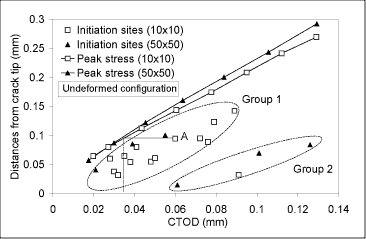Fig. 3. Initiation site and peak stress distances v's δ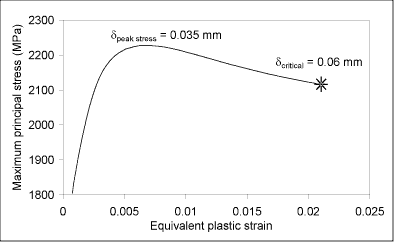Fig. 4. δ - εp history of data point A

Although final fracture is still stress-controlled, the necessary attainment of a critical plastic strain level invalidates Eqn. 1, which does recognize the necessity of plastic strain in the cleavage process through V p, but does not account for the determinant role of both its magnitude and build-up. The stress-only description of cleavage in Eqn. 1 inherently implicates that all potential nucleators are created at the onset of plasticity and that their number keeps constant with further plastic deformation.  Whilst this may have been a reasonable assumption for the steels investigated at the time, the present results indicate a significant difference for a more modern steel. They show that regions experiencing the highest tensile stresses may be less critical than regions with lower stresses but higher plastic strain levels. Interestingly, the invalidation of Eqn. 1 may explain the unrealistically high values that are sometimes obtained for the scale factor estimate Symbol.1. As the above results suggest, the actual risk of failure, P f, rises with increasing plastic strain as well as with stress. If Eqn. 1 is applied to describe the toughness scatter for the present material, the left-hand side of Eqn. 1, given by the experimental results, would rise more quickly than predicted by the right-hand side, which scales with stresses only. The data set of stresses would therefore wrongly appear to be not very scattered relative to the evolution of P f and this would be 'corrected' through an unrealistically large value of Symbol.1. Application of Eqn. 1 to the 10x10 mm 2 toughness results e.g. lead to Symbol.1 = 44.7, which is certainly an overestimation if one believes the last argument, yet of a magnitude often encountered in the literature. Inconsistent values of Symbol.1 in different geometries could also be explained by the different evolutions of the plastic strain according to the constraint level.

Such plastic strain effects should also invalidate Eqns. 2 and 3, since they are based on the same assumptions as Eqn. 1. The maximum likelihood (ML) method was used to fit the δc-results to Eqn. 2, with B 0 equal to 10 and 50 mm for Set 1 and 2 respectively (that is δ0-values are not size-corrected relative to any reference B 0 to be able to compare them directly). The two fitting curves are plotted in Figure 2. The ML estimates Symbol.2 and Symbol.3 were found to be equal to 2.2 and 0.055 mm for Set 1 and 1.7 and 0.050 mm for Set 2. The 95% confidence intervals for Symbol.2, Symbol.4, were [1.5, 3.2] and [1.2, 2.4] for Sets 1 and 2, respectively. These results are in good agreement with the theoretical value of 2 for α under SSY conditions and illustrate the point raised in the introduction, i.e. the ability of Eqn. 2 to describe toughness data even if Eqn. 1 produces suspicious results. Note also that the δ 0-values are not very different, which indicates a low size effect. A qualitative explanation for both points is proposed below.

## General applicability of closed-form SSY expressions

For every specimen examined on the SEM, a unique fracture origin was found, which is a prerequisite to the correct application of Eqns. 1 to 4 to describe the scatter in toughness results. However, considering the small specimen fracture tests, at least three different nucleation processes could be identified, namely: an intergranular failure type transforming to cleavage, a transgranular type with moderate plastic strain (Group 1) and a transgranular type with large plastic strain and microductility (Group 2). It was mentioned in the previous section that Eqns. 1 to 4 were not derived to account specifically for the change in plastic strain inside the plastic zone. Also, each equation is meant to address only one nucleating process at a time, not all of them (as was done in the preceding section), unless they are modified to do so. Here, the choice is made to address only the δc-values originating from the main nucleation mechanism, i.e. these of Group 1. The specimens that were not analyzed on the SEM are assumed to belong to Group 1, given that their δc-values fall in its range. A fit to Eqn. 2 of these δc-values only gives the following ML estimates: Symbol.2 = 2.72, Symbol.3 = 0.056 and Symbol.4 = [1.87, 3.94] for the 10x10 mm 2 specimens, Symbol.2 = 2.46, Symbol.3 = 0.041 and Symbol.4 = [1.67, 3.63] for the 50x50 mm 2 specimens. Once again, the estimated values for α approach the theoretical value of 2, but the size effect is this time more apparent.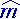Symbol.1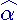Symbol.2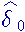Symbol.3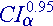Symbol.4

Following the discussion in the previous section, the above verification of Eqn. 2 is clearly not due to the evolution of the stress field in the SENB specimens alone. An alternative explanation is proposed in terms of a dual stress - plastic strain criterion. The model retains the fundamental assumptions that cleavage follows a weakest-link principle, is stress-controlled and that plastic flow is necessary for nucleation. To simplify, it is assumed that nucleation is controlled only by plastic strain and that propagation is controlled only by tensile stress. It is considered here that the material fractures at a certain critical tensile stress, constant over the range of present testing temperatures, i.e. the scatter is expected to be due only to the initiators location and plastic strain. There is experimental evidence showing that the number of nucleated microcracks increase with plastic strain and decreasing temperature. [9-11] The number of cracked carbides was found to increase proportional with plastic strain by Gurland  and Brindley,  results approaching proportionality were also obtained by Lindley et al.  At some temperatures, interpolation by a power law was more appropriate.  It is considered here that, for a small range of critical plastic strain values, at a given temperature, a linear relationship can be used. The influence of temperature will be inferred later. The last assumption is that if a fresh nucleated microcrack is not propagated immediately, that is if the tensile stress is less than the critical tensile stress, <σcrit, it blunts and will not be able to contribute to failure anymore. The cumulative cleavage failure probability can then be written as: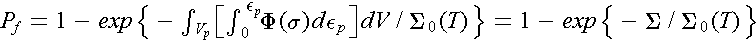where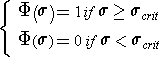(5)

εp is the equivalent plastic strain, Σ0(T) is a scaling factor dependent on temperature so that P f = 63.2% if Σ = Σ0(T), T is the test temperature. Comparison with Eqn. 2 shows that under SSY, this occurs if δ = δ0 (where δ 0 is no size-corrected, i.e. B = B 0). The double integral Σ, representing the risk of failure, increases in proportion to the plastic zone and the plastic strain. Note that only the fraction of ε p, which was coincident with a stress above the critical value ( σ ≥, σ crit is integrated, such that only the nucleated microcracks capable of propagation are considered. Σ 0(T) is introduced as a material parameter, which accounts for the effect of temperature on the microcracks nucleation rate. Σ 0(T) is therefore expected to decrease with temperature. Σ was calculated numerically from FE results, at -140°C for the 10x10 mm 2 geometry and at -130, -140, -150 and -196°C for the 50x50 mm 2 geometry. σ crit was chosen equal to 1800 MPa and only ε p > 0.2% data were integrated. The Σ-values are plotted against CTOD in Figure 5: it can be shown that they are proportional to δ 2. Assuming the model is correct, this would explain why Eqn. 2 is systematically reasonably verified, while Eqn. 1 may not apply. The values larger than 2 for the α-estimates obtained here may be due to the use of the two-parameter Weibull distribution ( δ min = 0).  Σ 0 was calculated for the 10x10 mm 2 geometry at -140°C, with δ 0 equated to the ML estimate Symbol.3 = 0.056 determined from the δ c-values: it was found equal to 0.022 mm 3. Knowing this value, Symbol.3 for the 50x50 mm 2 geometry tested at -140°C can then be inferred from Figure 5 and is equal to 0.024 mm. Using Eqn. 2 with α equal to the theoretical value of 2, the model predicts a size effect of (0.056/0.024) 2 = 5.44, which is only just higher than the expected 5-fold increase. The difference may be explained by the slightly higher triaxiality developed in the 50x50 mm 2 geometry. The next step consists of predicting the toughness scatter at other temperatures (for specimens failing by the same initiation process). As the evolution of Σ 0(T) with temperature is not known, it is interesting to predict the expression for Σ 0(T), assuming the model is valid over the range of testing temperatures. CTOD measurements at -150 and -196°C were available for the 50x50 mm 2 geometry: all δ c-values were considered to belong to Group 1's nucleation type and the calculated ML estimates for δ 0 were respectively 0.014 and 0.005 mm. This enabled the determination of Σ 0(T) at -130, -150 and -196°C, and it was found 0.045, 0.011 and 0.0036 mm 3 respectively ( Figure 5). Let Κ(T) be a temperature correction factor so that Κ(T) X Σ0 (T) = Σ0 (T 0) = constant. Here, T 0 is arbitrarily fixed to -130°C, thus κ(- 130 0C) = 1 and Σ 0(T 0) = 0.045. It can be shown that, when plotted against temperature, the four values of Κ(T) at -130, -140, -150 and -196°C are approximately aligned, increasing with decreasing temperature. A linear interpolation gives κ(T) ≈ κ(T) - 0.174(T - T0), for T ≤ TT0. Figure 6 shows the lower-shelf toughness curves for P f equal to 5, 63.2 and 95% predicted from Eqn. 5 where Σ 0(T 0) is replaced by 0.045/[1-0.174(T+130)]. The good agreement with the experimental results is expected since Κ(T) was calculated to tune the model to the results. Note that the two 50x50 mm 2 censored specimens (that belonged to Group 2) lie well above the 95% toughness curve. The next stage to valid the present proposal would be to determine the expression for Κ(T) from direct microcracks nucleation rate measurements.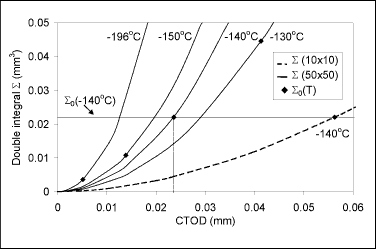Fig. 5. Temperature and size effects on Σ-values v's δ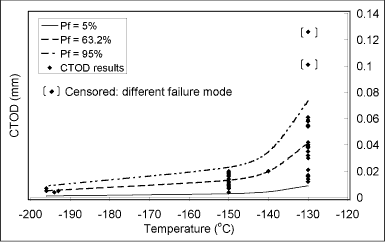Fig. 6. Lower-shelf toughness predictions

## Conclusion

This work has clearly unveiled the existence of a dual stress/plastic strain criterion for cleavage fracture in Grade 450EMZ. This invalidates the stress-only description that is commonly used to derive the theoretical probability of fracture by cleavage in steel. It has been shown that discounting the effect of plastic strain on the risk of failure explains the inconsistent and/or unrealistic values that are sometimes obtained for the shape factor m when using Eqn. 1. Classical expressions for assumed SSY conditions such as Eqn. 2 remained valid to describe the distribution of Grade 450EMZ toughness values, but it is suggested that this is because they are able to indirectly address the effects of plastic strain on cleavage fracture. An alternative expression has been developed which allows for the effect of plastic strain. This shows potential to accurately predict fracture toughness transition.

## Acknowledgements

Thanks are due to Professors A. H. Windle FRS and D. J. Fray FREng for the provision of laboratory facilities and TWI for the mechanical testing. The work reported here has been carried out under the PTP (Postgraduate Training Partnership) scheme which is funded by DTI and EPSRC.

## References

1. Beremin, F. M. (1983). Met. Trans. A, 14A, 2277-2287.
2. Wallin, K. (1984). Eng. Fract. Mech., 67, 1085-1093.
3. Anderson, T. L., Stienstra, D. and Dodds, R. H., Jr. (1994). In: Fracture Mechanics: Twenty-Fourth Volume, ASTM STP 1207, pp. 186-214, Landes, J. D., McCabe, D. E. and Boulet, J. A. M. (Eds). American Society for Testing and Materials, Philadelphia.
4. Pineau, A. (1992). In: Topics in Fracture and Fatigue, pp. 197-234, Argon, A. S. (Eds). Springer-Verlag, New York.
5. Brocks, W. (1996). In: Proceedings of the 11 th European Conference on Fracture, Poitiers, France. pp. 1-4, Petit, J et al. (Eds), EMAS, U.K.
6. Gao, X., Ruggieri. C. and Dodds, R. H., Jr. (1998). Int. Jour. Frac., 92, 2, 175-200.
7. Bordet, S. R., Karstensen., A. D., Wiesner, C. S. and Knowles, D. M. (submitted to Comp. Mat. Sci.).
8. Chen, J. H., Wang, G. Z., Wang, Z., Zhu, L. and Gao, Y. Y. (1991). Met. Trans. A, 2287-2296.
9. Gurland, J. (1972). Acta Met., 20, 735-741.
10. Brindley, B. J. (1970). Acta Met., 18, 325-329.
11. Lindley, T. C., Oates, G. and Richards, C. E. (1970). Acta Met., 18, 1127.